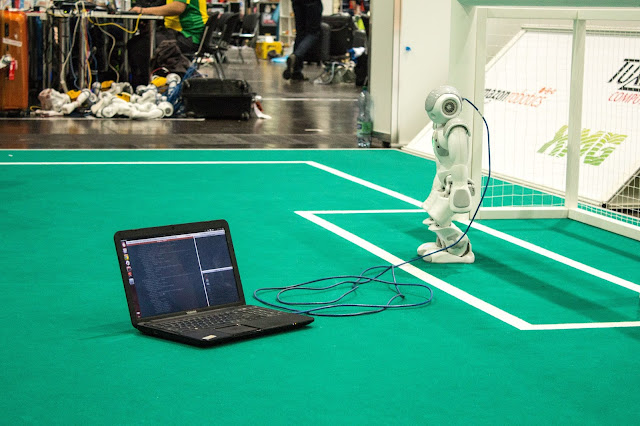## What's neural network?

We'll look at neural networks in this part.
Perceptrons, neural network architecture, convolutional neural networks, and recurrent neural networks are all covered in this section.
Recently, neural networks have become term, particularly in the context of the deep learning revolution that has occurred in recent years.
The neural network idea was originally proposed in the 1950s, and commercial applications began to appear in 1962.
perceptron is the most basic neural network.
perceptron is single_layer neural network that employs set of input characteristics to make decisions.
For instance, X1 to Xn.
These are all feature vectors, with X1 representing the cost and Xn representing the ratings.

The objective in this scenario may be to determine whether or not the client would buy something based on the inputs.

The bias term, which functions similarly to an intercept in linear regression models, is added to the input characteristics.
Actually, all of those characteristics are integrated in the same way as they are in linear regression.
As result, we get linear combination of features from the input feature, space, and the intercept.
We'll apply an activation function after we've obtained the linear combination.
This activation function is typically non_linear and is very dependent on the task at hand.
In this example, our response variable is binary, with one indicating purchase and zero indicating decision not to purchase product.
As we saw in the last section, the sigmoid function is logical approach to apply the activation function.

The perceptron is very basic network.
There is just one layer, which is made up of the sum of the linear inputs as well as the activation function, all of which are connected to the output.
Perceptrons were first proposed long time ago, and throughout time, more layers have been added to the perceptron.
For example, we have the input layer, the original layer, and several hidden layers in this image.
It becomes neural network if we have several layers.
Because the neural network topology is fairly complex, neural networks generally include many layers and many nodes inside each layer.

Neural networks are notoriously difficult to comprehend due to the large number of parameters in the model.
Because the topology of neural network may be intricate, and the number of parameters to be estimated is high, neural networks are also expensive to train.
There are several neural network frameworks in Scikit_learn that we may utilise.
However, since the deep learning revolution, individuals have been creating new frameworks for designing, training, estimating, and implementing neural networks.

As result, several layers have been created, with numerous nodes inside each layer, allowing input data to be considerably bigger than previously.
Because the training data for neural networks is so big and of such high quality, it may be quite beneficial.
MX net, TensorFlow, Cafe, and PyTorch are among of the most popular deep learning frameworks today.
These come from variety of places, but they can all be accessible using Python.
We can quickly build, train, fit, and implement neural network for any deep learning framework using Python.
Convolutional Neural Networks are type of neural network that is particularly effective for image processing.

The input to convolution neural network is either picture or sequence image that has been delayed.
To extract local characteristics from picture, we use kernels as filters.
We have the input picture in this example, and we're using filters to convolve with it to generate the next layer.
Depending on how many filters we're employing, the output from the convolutional layer will have different layers or channels, one in this example.
The pooling layer is another idea in convolutional neural networks.
You may wish to lower the size of the bit once you have certain output.
We can utilise either maximum pooling or average pooling to accomplish this.
By choosing the maximum of the two by two or the average of the two_by_two, we may reduce to just single scalar.
The pooling layer is essentially technique of dimension reduction.
Convolutional neural networks contain lot of layers and lot of dimensions, so you have lot of layers and lot of dimensions.
For better convergence, we need to minimise the amount of the data.
We can add few different layers to the convolution neural networks, but at the end of the day, we'll transform the tensor to vector and turn it into fully_connected layer.
To connect to the output, the completely connected layer will be used.

Typically, the output is certain category of the graph or the picture it includes.
This image's output may, for example, be digit zero.
We have lot of nice labelled data thanks to the training procedure.
We can use convolutional neural networks to try to figure out the optimal number of filters and filter variance that will give us near_human_level picture recognition accuracy.
In this instance, we can obtain neural near_human level of accuracy for handwritten digit recognition.
Recurrent Neural Networks are another form of neural network.
The input data is generally independent for the feed_forward neural network and the convolutional neural network.
The dependent structure among various input observations is impossible to represent using neural network.
However, when it comes to time, data sharing, or any other natural language processing or translation application, the order in which data is entered matters lot.
The recurrent neural network is the best option when the data contains sequential or time-sharing characteristics.

We can see the input layer, output layer, and all of the hidden layers in this high_level conceptual representation of recurrent neural network.
There are set of letters in the input to this recurrent neural network, but they have significance as sequence.
So, until we have sequential relationship between them, each individual word, each individual character, doesn't signify anything.
Information does not travel in single way during the training process.
The information flow is really repeated in multiple sequences as it propagates via other nodes.
The input layer and output layer are really coupled using this recurrent neural network in the end result.
I'm Kunal Katke, and appreciate you taking the time to read this.

##What is threshold function in neural network?

We'll look at the idea of threshold functions, different types of threshold functions, and logic gates in artificial neural networks in this blog.
One of the most important components of artificial neural networks is the threshold function.
The goal of threshold is to assess whether or not the neurons are triggered.
By computing the weighted sum and adding direction, threshold functions generally incorporate nonlinear characteristics into an artificial neural network.
This will assist us determine whether or not to stimulate certain neuron.
Threshold functions are commonly employed in biological neural network_based computing systems.
It's also utilised to measure the output of the output layer's neurons.
When we utilise neural networks to do categorization, we employ these quantified values.
Now, the threshold function is Boolean function that we use to see if the value of the input exceeds predetermined threshold, which is one of the activation functions commonly employed in artificial neural networks.
Let's look at some of the most common forms of threshold functions now that we've learned about the notion of threshold functions and how to use them.

Unit step, Sigmoid, Piecewise linear, Gaussian, and Linear are five types of threshold functions that may be used in artificial neural networks.
The output of unit step is set to one of two levels: more than or less than.
This function is often used to determine whether the total output exceeds or falls below predetermined threshold value.
The sigmoid threshold value, on the other hand, usually includes two extra functions: logistic function and tangential function.
From the standpoint of their range of values, these two functions are distinct.

The logistic function has value between and 1, while the tangential function has value between -1 and 1.
third form of threshold function is piecewise linear, in which the output is directionally proportional to the weighted output.
Gaussian functions are continuous curves with bell form.
Neuron levels can range from low to high.
The current level is interpreted based on how near the neuron input is picked after averaging to the identified value.
Finally, linear regression and the linear function are quite similar.

When we apply the linear activation function, the weighted aggregate inputs of neurons are converted into an output.
It is now critical to understand logic gates and how they may be used in artificial neural networks.
Logic gates can aid in the extraction of important values and models that can be used to construct or implement neural network models.
The use of AND, OR, and XNOR gates is depicted in the three example illustration pictures.
The application of the AND gate is shown in the first sample figure on the left.
We can see that when both input values are one, the output value is one.

The application of the OR gate is shown in the second example figure in the middle, and we can see that we obtain the output value one whenever one of the input values is one.
Finally, the application of the XNOR gate is shown in the third sample picture on the right.
And we can see that anytime both input numbers are the same, we receive the output value 1.
We may develop basic models for complicated artificial neural network scenarios by utilising these logic gates on neurons.
appreciate you taking the time to read this.